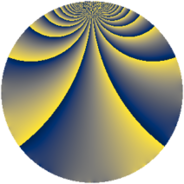# Properties

 Label 2850.2.tLevel $2850$ Weight $2$ Character orbit 2850.t Rep. character $\chi_{2850}(2501,\cdot)$ Character field $\Q(\zeta_{6})$ Dimension $256$ Sturm bound $1200$

# Learn more about

## Defining parameters

 Level: $$N$$ $$=$$ $$2850 = 2 \cdot 3 \cdot 5^{2} \cdot 19$$ Weight: $$k$$ $$=$$ $$2$$ Character orbit: $$[\chi]$$ $$=$$ 2850.t (of order $$6$$ and degree $$2$$) Character conductor: $$\operatorname{cond}(\chi)$$ $$=$$ $$57$$ Character field: $$\Q(\zeta_{6})$$ Sturm bound: $$1200$$

## Dimensions

The following table gives the dimensions of various subspaces of $$M_{2}(2850, [\chi])$$.

Total New Old
Modular forms 1248 256 992
Cusp forms 1152 256 896
Eisenstein series 96 0 96

## Trace form

 $$256q - 3q^{3} - 128q^{4} - q^{6} + 12q^{7} + q^{9} + O(q^{10})$$ $$256q - 3q^{3} - 128q^{4} - q^{6} + 12q^{7} + q^{9} - 18q^{13} - 128q^{16} - 18q^{19} - 30q^{22} - q^{24} - 6q^{28} + 33q^{33} + 12q^{34} + q^{36} - 10q^{42} + 42q^{43} + 3q^{48} + 284q^{49} - 18q^{51} + 18q^{52} + 44q^{54} - 28q^{57} - 38q^{61} - 20q^{63} + 256q^{64} - q^{66} + 60q^{67} + 3q^{72} + 8q^{73} + 24q^{76} + 30q^{78} - 42q^{79} + 57q^{81} - 6q^{82} + 60q^{87} - 114q^{91} - 10q^{93} + 2q^{96} - 30q^{97} + 29q^{99} + O(q^{100})$$

## Decomposition of $$S_{2}^{\mathrm{new}}(2850, [\chi])$$ into newform subspaces

The newforms in this space have not yet been added to the LMFDB.

## Decomposition of $$S_{2}^{\mathrm{old}}(2850, [\chi])$$ into lower level spaces

$$S_{2}^{\mathrm{old}}(2850, [\chi]) \cong$$ $$S_{2}^{\mathrm{new}}(57, [\chi])$$$$^{\oplus 6}$$$$\oplus$$$$S_{2}^{\mathrm{new}}(114, [\chi])$$$$^{\oplus 3}$$$$\oplus$$$$S_{2}^{\mathrm{new}}(285, [\chi])$$$$^{\oplus 4}$$$$\oplus$$$$S_{2}^{\mathrm{new}}(570, [\chi])$$$$^{\oplus 2}$$$$\oplus$$$$S_{2}^{\mathrm{new}}(1425, [\chi])$$$$^{\oplus 2}$$